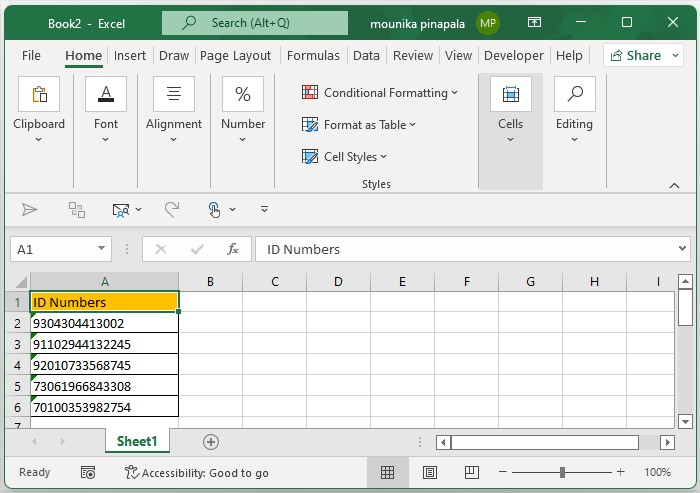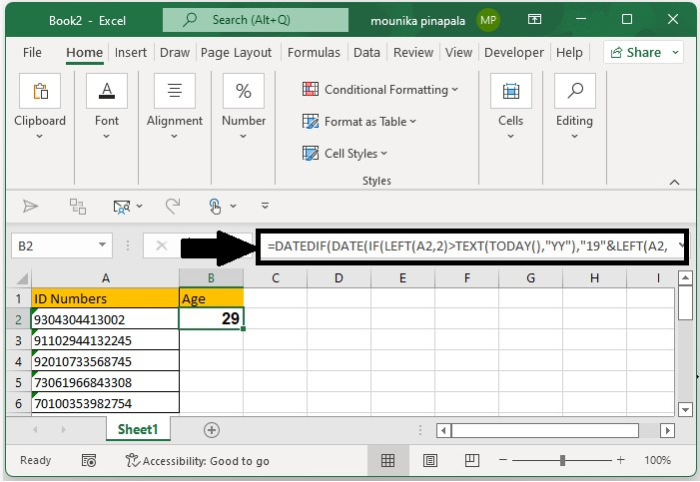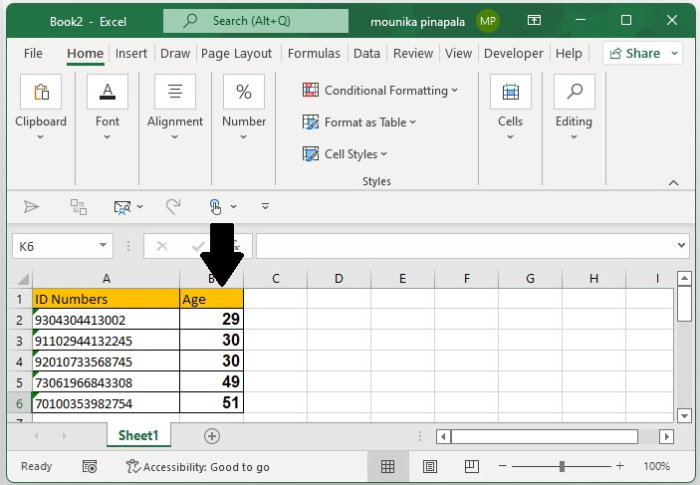# How to calculate age from ID number in Excel?

Let's consider that you have a list of ID numbers that contains 13 to 15 digits of numbers, and in those 13 to 15 digits, the first six digits are the date of birth of the candidate or a specific person, so that you have a list of ID numbers.

For instance, consider that you have an ID number of one person 9304304413002 in this Id first 6 digits is the date of birth 1993/04/30. From this ID number, how will you calculate the age? In this article, I will introduce the formula which is very easy that quickly finds out the age of a person from the given ID number.

Follow the steps given below to understand easily how to calculate the age from the ID number in the Excel sheet.

### Step 1

Open a Microsoft excel sheet and enter the ID numbers as shown in the below screenshots for your reference. You can change the ID numbers as per your wish or as per the given by your company.### Step 2

Now you need to select a blank cell and enter the below given formula to calculate the age from the given ID number. In our case, I will enter the formula in the cell B2 as shown in the below screenshot for your reference.

=DATEDIF(DATE(IF(LEFT(A2,2)>TEXT(TODAY(),"YY"),"19"&LEFT(A2,2),"20"&LEFT A2,2)),MID(A2,3,2),MID(A2,5,2)),TODAY(),"y")### Step 3

Now you need to drag the fill handle down till you want to calculate the age for the ID numbers given. Apply the formula and the ages will be calculated from the ID numbers as shown in the below screenshot for you reference.Note − In the above formula, A2 contains the list of ID numbers that you want to calculate the age. If the year is less than the present year, then it will be considered 20, otherwise 19 if the year is greater than the present year.

## Conclusion

In this tutorial, we used a simple example to demonstrate how you can calculate age from ID number in Excel.